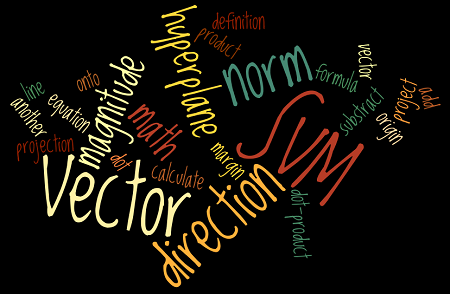## SVM - Understanding the math - Duality and Lagrange multipliers

This is the Part 6 of my series of tutorials about the math behind Support Vector Machines. Today we will learn about duality, optimization problems and Lagrange multipliers. If you did not read the previous articles, you might want to start the serie at the beginning by reading this article: an overview of Support Vector Machine. ... Read more

## SVM - Understanding the math - Convex functions

This is the Part 5 of my series of tutorials about the math behind Support Vector Machines. Today we are going to study convex functions. If you did not read the previous articles, you might want to start the serie at the beginning by reading this article: an overview of Support Vector Machine. How can we ... Read more

## SVM - Understanding the math - Unconstrained minimization

This is the Part 4 of my series of tutorials about the math behind Support Vector Machines. Today we are going to learn how to solve an unconstrained minimization problem. If you did not read the previous articles, you might want to start the serie at the beginning by reading this article: an overview of Support ... Read more

## SVM - Understanding the math - the optimal hyperplane

This is the Part 3 of my series of tutorials about the math behind Support Vector Machine.

If you did not read the previous articles, you might want to start the serie at the beginning by reading this article: an overview of Support Vector Machine.

Here is a quick summary of what we will see:

• How can we find the optimal hyperplane ?
• How do we calculate the distance between two hyperplanes ?
• What is the SVM optimization problem ?

## How to find the optimal hyperplane ?

At the end of Part 2 we computed the distance  $\|p\|$ between a point  $A$ and a hyperplane. We then computed the margin which was equal to $2 \|p\|$.

However, even if it did quite a good job at separating the data it was not the optimal hyperplane.

## SVM - Understanding the math - Part 2This is Part 2 of my series of tutorial about the math behind Support Vector Machines.
If you did not read the previous article, you might want to start the serie at the beginning by reading this article: an overview of Support Vector Machine.

In the first part, we saw what is the aim of the SVM. Its goal is to find the hyperplane which maximizes the margin.

But how do we calculate this margin?

## SVM = Support VECTOR Machine

In Support Vector Machine, there is the word vector.
That means it is important to understand vector well and how to use them.

Here a short sum-up of what we will see today:

• What is a vector?
• its norm
• its direction
• How to add and subtract vectors ?
• What is the dot product ?
• How to project a vector onto another ?

Once we have all these tools in our toolbox, we will then see:

• What is the equation of the hyperplane?
• How to compute the margin?

## Introduction

This is the first article from a series of articles I will be writing about the math behind SVM. There is a lot to talk about and a lot of mathematical backgrounds is often necessary. However, I will try to keep a slow pace and to give in-depth explanations, so that everything is crystal clear, even for beginners.

If you are new and wish to know a little bit more about SVMs before diving into the math, you can read the article: an overview of Support Vector Machine.

## What is the goal of the Support Vector Machine (SVM)?

The goal of a support vector machine is to find  the optimal separating hyperplane which maximizes the margin of the training data.

The first thing we can see from this definition, is that a SVM needs training data. Which means it is a supervised learning algorithm.

It is also important to know that SVM is a classification algorithm. Which means we will use it to predict if something belongs to a particular class.

For instance, we can have the training data below: